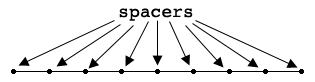SEARCH HOMEMath Central Quandaries & QueriesQuestion from Christopher: Ok I've got forty foot and 9 spacers one each end and 7 between what is the distance between the seven in the middleHi Christopher. I drew a diagram and assumed that the spacers are evenly spaced along the 40 feet.From the diagram the spacers divide the 40 feet into 8, equal size pieces. Hence each piece is $\large \frac{40}{8} \normalsize = 5$ feet in length.

PennyMath Central is supported by the University of Regina and The Pacific Institute for the Mathematical Sciences.